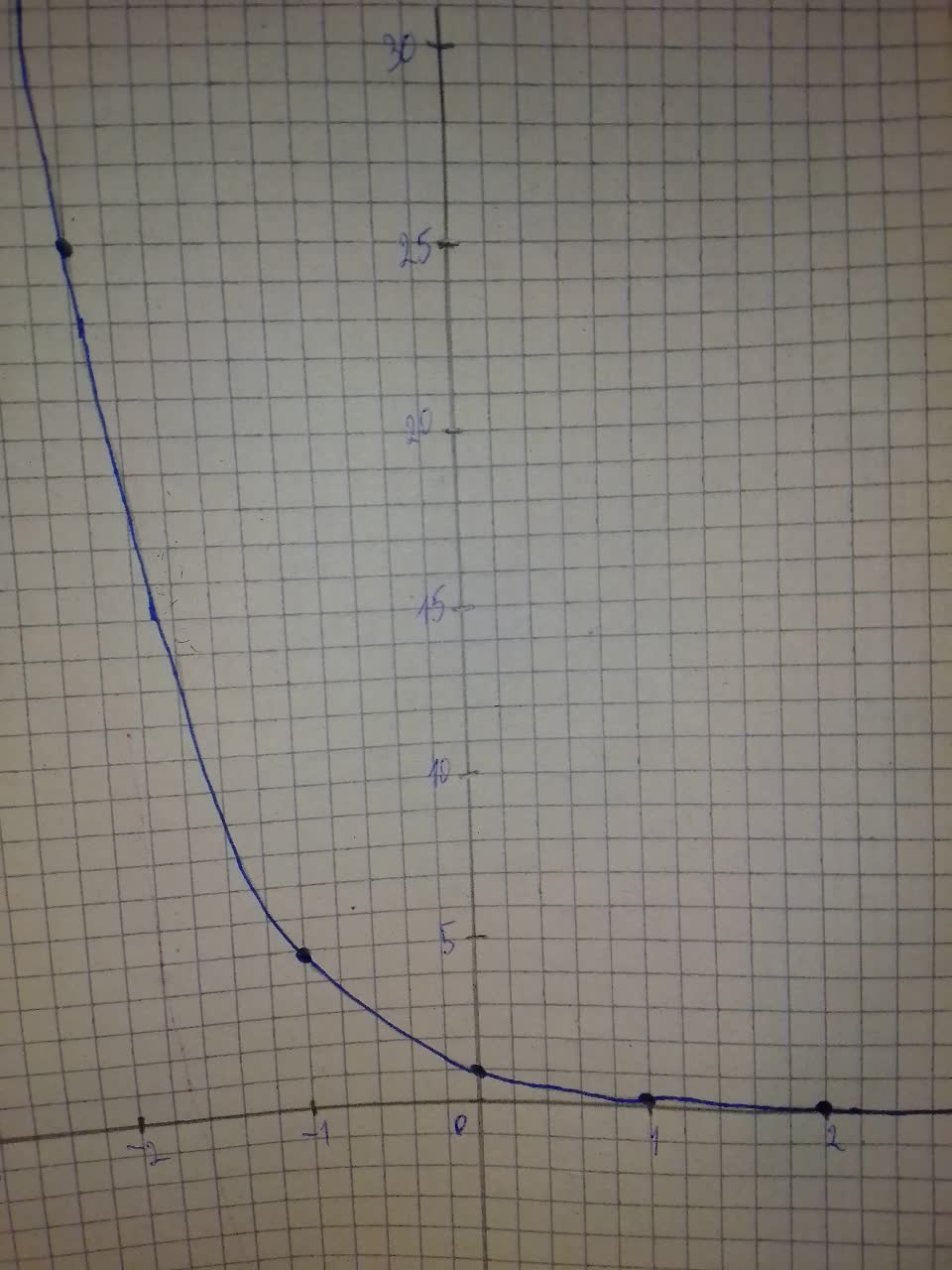Question# Determine whether the function represents exponential growth or exponential decay f(x)=(0.2)^{x}

Exponential growth and decay
ANSWEREDDetermine whether the function represents exponential growth or exponential decay. Identify the percent rate of change. Then graph the function.
$$\displaystyle{f{{\left({x}\right)}}}={\left({0.2}\right)}^{{{x}}}$$2021-08-01
Step 1 The function is an exponential decay since its base (which is 0.2) is less than 1.
$$\displaystyle{1}-{r}={0.2}$$
$$\displaystyle{r}={0.8}$$
$$\displaystyle{r}={80}\%$$
The rate of decay is about $$\displaystyle{80}\%$$
Step 2